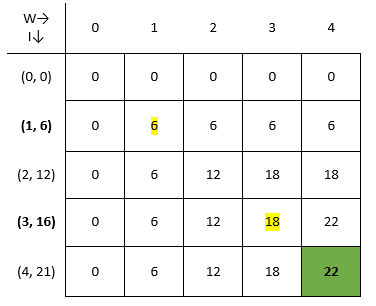# Solving 0/1 knapsack problem using dynamic programming

 Category: Data Structure And Algorithms Tags:Knapsack Problem Code Files

## Introduction

Knapsack problem is similar how a thief works, suppose a thief breaks in some shop having knapsack where he can carry only 4kg, now there are so many items up to 4kg but he has to pick up items which gives him maximum value, suppose items weight and their respective values are given like:

 Weight (Kg) 1 2 3 4 Value (\$) 6 12 16 21

## Implementation

We will solve this problem with dynamic programming because we can take benefit of memoization, suppose if we know what is the maximum profit if we have knapsack of capacity 2kgs then why to calculate same sub-problem when we have knapsack of 4kg(4kg knapsack problem again will be broken in subproblems like 2kg+2kg). For that we are going to create a class structure:

```public class KnapsackManager
{
int knapsackSize;
int[] _weights;
int[] _values;
int[,] results;

public KnapsackManager(int[] weights, int[] values, int size)
{
_weights = weights;
_values = values;
knapsackSize = size;
}

public int CreateSolution(){...}
}
```

Now we have set item's weight respective values and knapsack size using constructor. Now we have to implement CreateSolution method:

```/// <summary>
/// Calculates maximum profit
/// </summary>
/// <returns></returns>
public int CreateSolution()
{
results = new int[_weights.Length + 1, knapsackSize + 1];

for (int i = 0; i < _weights.Length; i++)   // item 1 to n
{
for (int j = 1; j <= knapsackSize; j++) //weight 1 to m
{
if (_weights[i] > j)              //if item weight is less than current index's weight
{
results[i + 1, j] = results[i, j];    //if item weight is grater than knapsack capacity
}

else
{
if (results[i, j] > (_values[i] + results[i, j - _weights[i]]))
{
//if previously calculated value only is grater
results[i + 1, j] = results[i, j];
}
else
{
//if including current item gives more value
results[i + 1, j] = _values[i] + results[i, j - _weights[i]];
}
}
}
}
return results[_weights.Length, knapsackSize]; // index (n, m) will be max value
}```

Above code is having two loops, outer loop is going through each item and inner loop going through 1 to knapsack size. Here we can see if knapsack size is less than item weight than we can’t take that item and we have to take whatever was there previously. And if knapsack is having equivalent or more capacity we can try with current item or current item with combination of other items, whichever combination gives more value we will keep that in results. And obviously last indexes of matrix (result[items, knapsack_size]) will give max of value which is our solution. Above solution will form result matrix like:Above you can see W is weight from 0 to knapsack size and I is items displayed in format of (weight, value). Green box is our solution. It goes like, for item 1 and weight 1 it checks we can take item 1 if size of knapsack is 1 or more so first element in matrix calculates (value of item 1 + maximum value at weight (1-1) last calculated) = (6+0) = 6. If in same row(item1 row) we go for weight 2 column(which is actually knapsack size, we are creating sub-problems) we again calculate (value of item 1 + maximum value at weight (2-1) last calculated) = (6+0) = 6. if we talk about item2 and weight 3 there we can see value of item2 is 12 and after putting it in knapsack we still left with weight 1 (3-2 = 1), so last time measured maximum weight was 6 (see in column w=1 and row item = 1) for weight 1 so maximum would be (12+6) = 18 we shall keep in results and so on. We can execute this method:

```static void Main(string[] args){    KnapsackManager demo = new KnapsackManager(new int[] { 1, 2, 3, 4 }, new int[] { 6, 12, 16, 21 }, 4);    Console.WriteLine("Solution is: " + demo.CreateSolution());    Console.ReadLine();}
```

And output will be:

Solution is: 22

## Analysis

If we think about iterations of loops clearly it is m.n but we are saving calculation time using memoization of sub-problems. Complexity will be O(m.n) but if we wouldn’t have used memoization it would be Ω(m.n).

 Like0 PeopleNikhil Joshi Ceo & Founder at Dotnetlovers Atricles: 146 Questions: 16 Given Best Solutions: 16 *

Login via:x